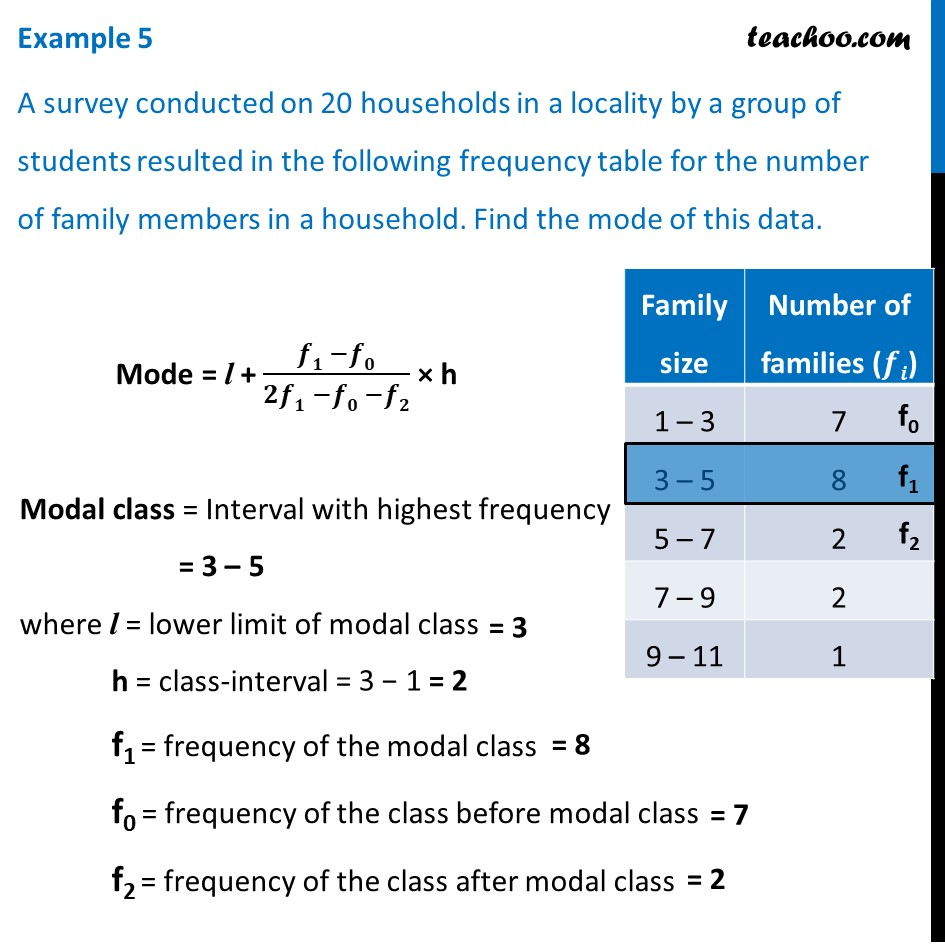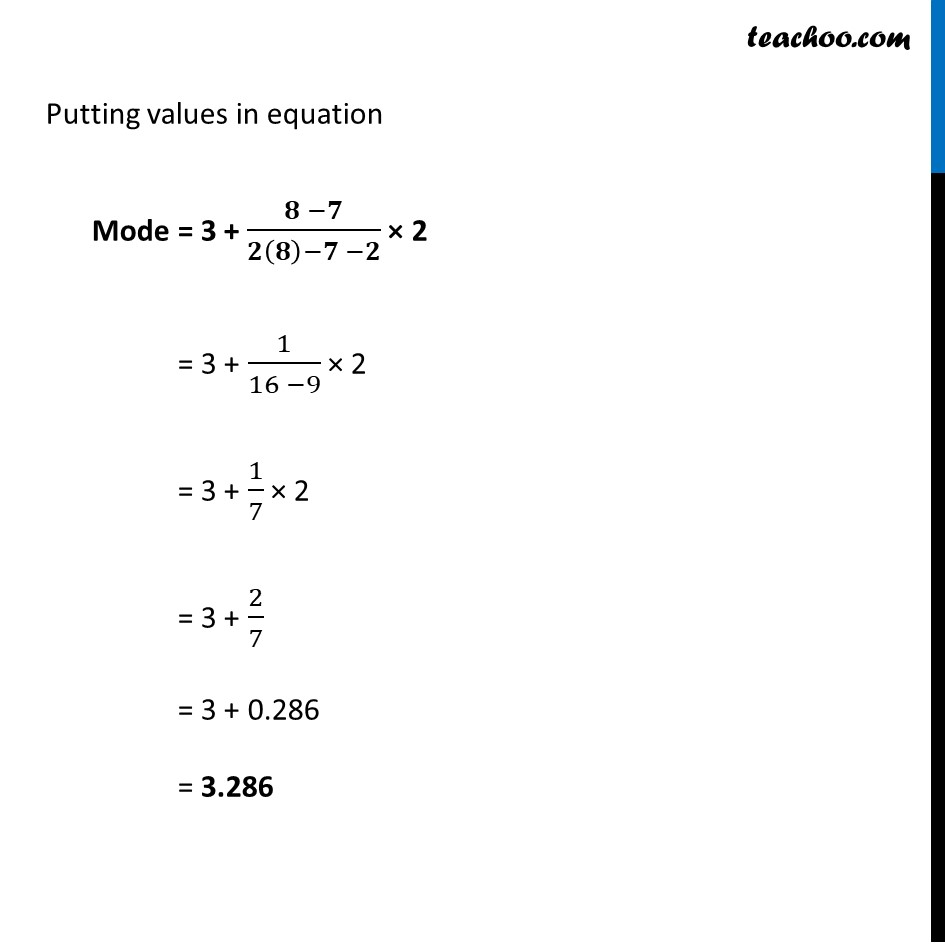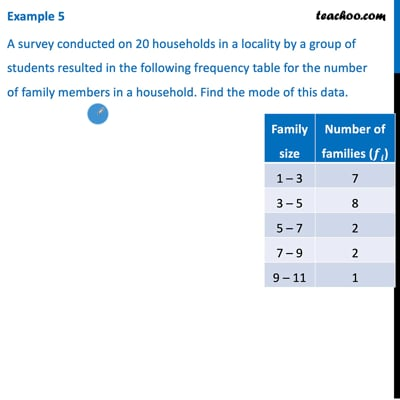Examples

Chapter 14 Class 10 Statistics
Serial order wiseThis video is only available for Teachoo black users

Solve all your doubts with Teachoo Black (new monthly pack available now!)

### Transcript

Example 5 A survey conducted on 20 households in a locality by a group of students resulted in the following frequency table for the number of family members in a household. Find the mode of this data. Mode = l + (𝒇𝟏 −𝒇𝟎)/(𝟐𝒇𝟏 −𝒇𝟎 −𝒇𝟐) × h Modal class = Interval with highest frequency = 3 – 5 where l = lower limit of modal class h = class-interval f1 = frequency of the modal class f0 = frequency of the class before modal class f2 = frequency of the class after modal class Putting values in equation Mode = 3 + (𝟖 −𝟕)/(𝟐(𝟖)−𝟕 −𝟐) × 2 = 3 + 1/(16 −9) × 2 = 3 + 1/7 × 2 = 3 + 2/7 = 3 + 0.286 = 3.286